$B7DXf5A=NBg3X(B 2010$BG/EY(B $B=U3X4|(B # $B%7%9%F%!&%=%U%H%&%'%"(B System Software / Operating Systems

2010$BG/EY=U3X4|!!2PMKF|(B2$B;~8B(B
$B2JL\%3!<%I(B: 60730 $B3+9V>l=j!'(BSFC
$B $BC4Ev(B: Rodney Van Meter
E-mail: rdv@sfc.keio.ac.jp

## Outline

• Today's Pictures
• Concurrent Data Structures
• Structure of a research proposal
• Performance measurement statistics
• Memory management
• Virtual memory (introduction)

## Today's Pictures

Ken Eason's "Fragmentation"

## Upcoming CI Seminars

CI Seminar Web Page

## Structure of a Research Project Proposal

Notes at substantially updated.

## Performance Measurement Statistics

• Gaussian normal distribution
• Long tail and other distributions
• Clock granularity
• Error Bars
• Fitting and statistical software
There really is no substitute for learning the mathematics of probability and statistics if you want to do performance analysis of computer systems, and if you're in either research or development, you probably do want to measure the performance of your work at some point. However, this class is not the right place to do comprehensive statistics. We'll take a quick look at some of the things you might expect, though.

### Normal Gaussian Distribution

gnuplot's norm() function seems to give the cumulative distribution. So, to do a poor man's derivative to get a Gaussian,

gnuplot> delta=0.01
gnuplot> g(x) = (norm(x+delta)-norm(x))/delta
gnuplot> set title "Gaussian Normal Density"
gnuplot> plot [-4:4] [0:0.5] g(x) notitle lw 3
gnuplot> set term post eps "Helvetica" 24
gnuplot> set out "normal.eps"
gnuplot> replot
[rdv@localhost systems-software]$file normal.eps normal.eps: PostScript document text conforming at level 2.0 - type EPS [rdv@localhost systems-software]$ convert -size 720x504 -resize 720x504 normal.eps normal.png
[rdv@localhost systems-software]$file !$
file normal.png
normal.png: PNG image data, 720 x 504, 16-bit/color RGB, non-interlaced
[rdv@localhost systems-software]$display !$
display normal.png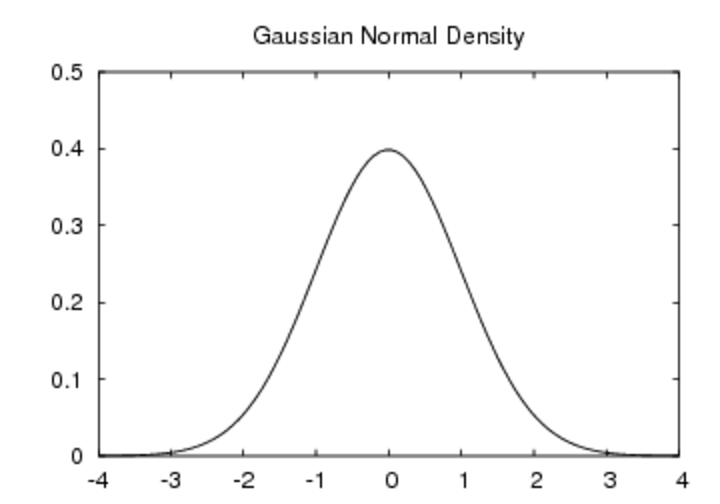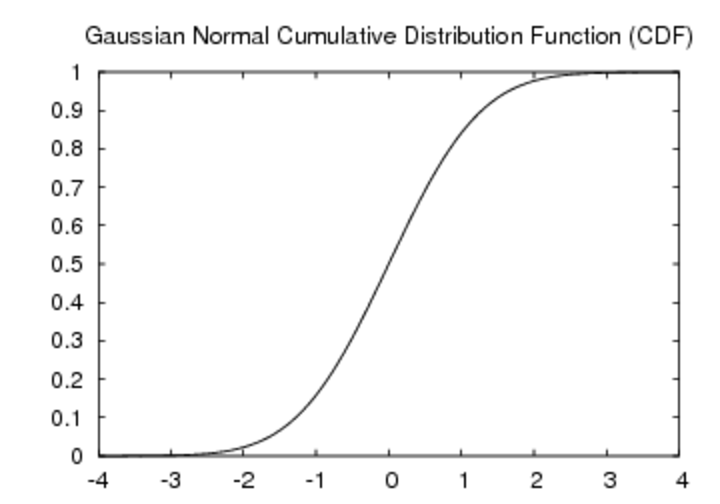The normal distribution is a continuous function; its discrete counterpart is the Poisson distribution.

### Other Distributions

As I have mentioned, other distributions of times are possible. Two of the most commonly seen ones are a long-tailed distribution and a bimodal distribution. Cauchy is the name of one form of long-tailed distribution. Long-tailed distributions are common on the Internet as a description of e.g. connection lifetimes.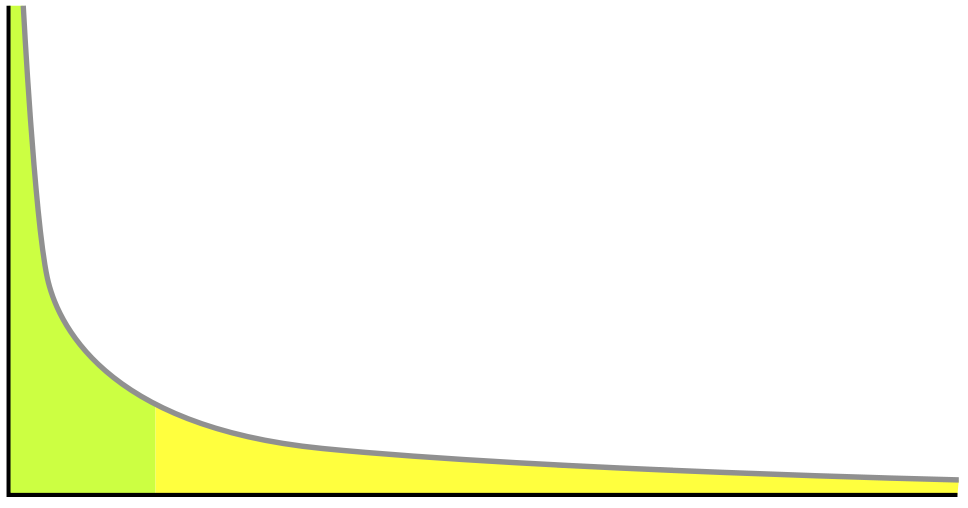### Clock Granularity Artifacts

In order to measure something very short, you need to do
startclock();
for ( i = 0 ; i < NUMREPS ; i++ )
do_short_operation();
stopclock();

for some value of NUMREPS like 100 or 1000. This still doesn't tell you about the exact distribution of the time for the short operations, but it can tell you about the mean.

A few of you have already hit on using the Intel processor Time Stamp Counter (TSC). That's an excellent idea, but it does have drawbacks:

• It's not portable
• If clock frequency changes, it's not wall clock time
• It can't account for multitasking

### Error Bars

All data should have error bars. The error bars may be the standard deviation, 90% confidence interval, 95% confidence interval, or, in rare cases, the high and low values.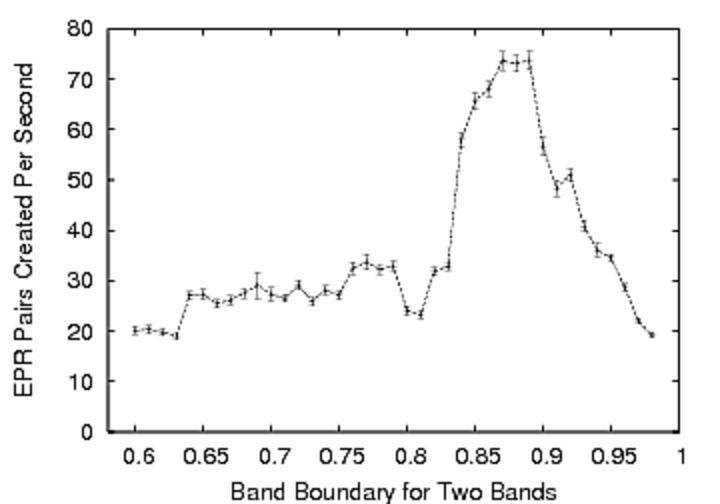### Linear Fit

There are many packages for doing fitting and other statistics available on the Internet and in any sort of mathematics-oriented language, such as Mathematica, Matlab or Octave. My personal recommendation is that you use John Heidemann's JDB to hold your experimental results and do the processing and fitting for you, but you are free to do whatever you want.

Recently, for a project, I adapted the function gsl_fit_linear for some code. The adaptation was actually a hassle, so I don't recommend you do it, but for what it's worth, here's the code itself from the GNU Scientific Library (GSL).

/* Fit the data (x_i, y_i) to the linear relationship

Y = c0 + c1 x

returning,

c0, c1  --  coefficients
cov00, cov01, cov11  --  variance-covariance matrix of c0 and c1,
sumsq   --   sum of squares of residuals

This fit can be used in the case where the errors for the data are
uknown, but assumed equal for all points. The resulting
variance-covariance matrix estimates the error in the coefficients
from the observed variance of the points around the best fit line.
*/

int
gsl_fit_linear (const double *x, const size_t xstride,
const double *y, const size_t ystride,
const size_t n,
double *c0, double *c1,
double *cov_00, double *cov_01, double *cov_11, double *sumsq)
{
double m_x = 0, m_y = 0, m_dx2 = 0, m_dxdy = 0;

size_t i;

for (i = 0; i < n; i++)
{
m_x += (x[i * xstride] - m_x) / (i + 1.0);
m_y += (y[i * ystride] - m_y) / (i + 1.0);
}

for (i = 0; i < n; i++)
{
const double dx = x[i * xstride] - m_x;
const double dy = y[i * ystride] - m_y;

m_dx2 += (dx * dx - m_dx2) / (i + 1.0);
m_dxdy += (dx * dy - m_dxdy) / (i + 1.0);
}

/* In terms of y = a + b x */

{
double s2 = 0, d2 = 0;
double b = m_dxdy / m_dx2;
double a = m_y - m_x * b;

*c0 = a;
*c1 = b;

/* Compute chi^2 = \sum (y_i - (a + b * x_i))^2 */

for (i = 0; i < n; i++)
{
const double dx = x[i * xstride] - m_x;
const double dy = y[i * ystride] - m_y;
const double d = dy - b * dx;
d2 += d * d;
}

s2 = d2 / (n - 2.0);        /* chisq per degree of freedom */

*cov_00 = s2 * (1.0 / n) * (1 + m_x * m_x / m_dx2);
*cov_11 = s2 * 1.0 / (n * m_dx2);

*cov_01 = s2 * (-m_x) / (n * m_dx2);

*sumsq = d2;
}

return GSL_SUCCESS;
}


Sometimes, a line is a good fit for only part of your total data. Sometimes, a different line will fit a later portion of your data; such a case is called a multi-linear fit.

## Memory Management

• Goals of memory management
• Pointers and memory addresses
• Multi-level memory hierarchy
• Memory map
• Basic techniques for dynamic allocation/deallocation
• Simple multiprogramming memory management

### Goal of Memory Management

The primary goal of memory management is to support dynamic growth and shrinking of resources. Why?

• programs may not be able to allocate all needed memory at compile and program load time
• safe sharing of memory
(capacity management for correctness and fairness, and security)

### Multi-level Memory Hierarchy

Most computer systems support a multi-level memory hierarchy:
• Registers
• Cache (sometimes multi-level itself)
• Main memory
• Disk
• (Tape, in some supercomputing systems)
where all of the levels are managed by the compiler and operating system together to be transparent to the application programmer, except for performance. Sometimes the transparency is partially aided by hardware, as in the case of cache memory.

Four questions on the memory hierarchy:

• Where can the item be placed in memory? (placement)
• How is the memory found? (naming)
• When there's not enough memory, what gets removed or replaced? (replacement)
• What happens on write? (write strategy)

### Pointers and Memory Addresses

If you program in C at all, you should be familiar with pointers by now, but let me go over it quickly...

### Memory Map

The most important concept tool for visualizing the location of data is the memory map. Memory maps can be drawn with high addresses at the top or the bottom.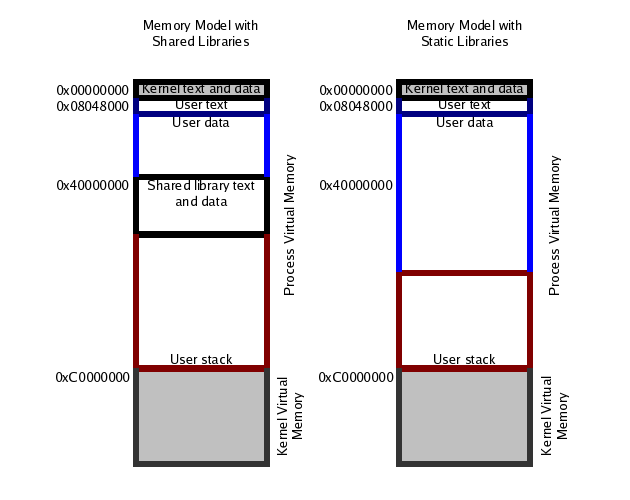(Image from NCSU.)

### Basic Techniques

The most important task of the memory manager is to keep track of which memory is free and which is allocated. That task can be done using bitmaps or linked lists.

Sometimes, memory is wasted due to a process known as fragmentation. Fragmentation occurs when various objects are created and deleted, leaving behind holes in the memory space. The memory manager's job is to see that applications can always get the memory they need, by using an algorithm that minimizes fragmentation and keeps holes under control.

Several different algorithms can be used to assign memory to the next request that comes in:

• first fit
• best fit
• worst fit
• buddy system
Probably all operating systems internally use a technique called quick fit, in which separate lists are maintained for commonly-requested sizes. 4K, 1500, and 128 bytes are common sizes. (Actually, it would be more correct to say that a multi-level memory manager is at work here; the network subsystem calls the primary memory manager to allocate a large chunk of memory, which it then manages itself and divides up into smaller chunks for buffers for various things.)

[rdv@dhcp-143-236 ~]$more /proc/buddyinfo Node 0, zone DMA 2 4 3 4 5 4 2 2 3 1 1 Node 0, zone Normal 242 110 156 111 78 43 20 7 7 4 3 Node 0, zone HighMem 2 0 0 1 1 1 0 0 0 0 0  ## Simple Multiprogramming Memory Management • Base and limit registers, as in the Cray-1. • Complete swapping of processes. With base and limit registers, the base register is added to every memory request, and checked against the limit register. Thus, when the OS schedules a different process, the only those two registers have to change. The original form of multiprogramming actually involved swapping complete processes into and out of memory, to a special reserved area of disk (or drum). This approach allowed each process to act as if it owned all of the memory in the system, without worrying about other processes. However, swapping a process out and in is not fast! ## Introduction to Virtual Memory • Each process has its own address space. • Page tables are maintained by the OS and used by the hardware to map logical addresses to physical addresses. • Linux page tables. Finally, we come to virtual memory $B!J2>A[5-O?!K(B. With virtual memory, each process has its own address space. This concept is a very important instance of naming. Virtual memory (VM) provides several important capabilities:

• VM hides some of the layers of the memory hierarchy.
• VM's most common use is to make memory appear larger than it is.
• VM also provides protection and naming, and those are independent of the above role.
In most modern microprocessors intended for general-purpose use, a memory management unit, or MMU, is built into the hardware. The MMU's job is to translate virtual addresses into physical addresses.

## Page Tables

Viritual memory is usually done by dividing memory up into pages, which in Unix systems are typically, but not necessarily, four kilobytes (4KB) each. The page table is the data structure that holds the mapping from virtual to physical addresses. The page frame is the actual physical storage in memory.

The simplest approach would be a large, flat page table with one entry per page. The entries are known as page table entries, or PTEs. However, this approach results in a page table that is too large to fit inside the MMU itself, meaning that it has to be in memory. In fact, for a 4GB address space, with 32-bit PTEs and 4KB pages, the page table alone is 4MB! That's big when you consider that there might be a hundred processes running on your system.

The solution is multi-level page tables. As the size of the process grows, additional pages are allocated, and when they are allocated the matching part of the page table is filled in.

The translation from virtual to physical address must be fast. This fact argues for as much of the translation as possible to be done in hardware, but the tradeoff is more complex hardware, and more expensive process switches. Since it is not practical to put the entire page table in the MMU, the MMU includes what is called the TLB: translation lookaside buffer.

## Linux Page Tables

PGD is the page global directory. PTE is page table entry, of course. PMD is page middle directory.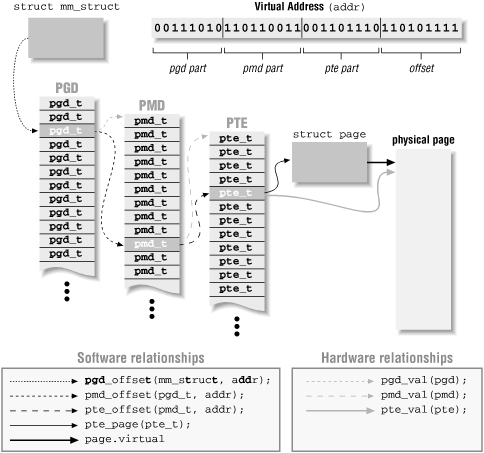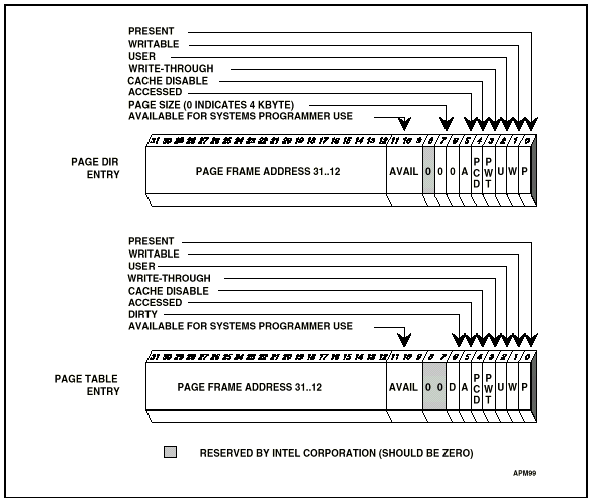(Images from O'Reilly's book on Linux device drivers, and from lvsp.org.)

We don't have time to go into the details right now, but you should be aware that doing the page tables for a 64-bit processor is a lot more complicated, when performance is taken into consideration.

Linux uses a three-level page table system. Each level supports 512 entries: "With Andi's patch, the x86-64 architecture implements a 512-entry PML4 directory, 512-entry PGD, 512-entry PMD, and 512-entry PTE. After various deductions, that is sufficient to implement a 128TB address space, which should last for a little while," says Linux Weekly News.

#define IA64_MAX_PHYS_BITS      50      /* max. number of physical address bits (architected) */
...
/*
* Definitions for fourth level:
*/
#define PTRS_PER_PTE    (__IA64_UL(1) << (PTRS_PER_PTD_SHIFT))



## Paging

Next week, we will discuss the process of paging, where parts of memory are stored on disk when memory pressure is high.

## Homework

This week's homework:

1. Experimentally construct a rough memory map for an application on your operating system.
1. Write a program that prints out (in hexadecimal) the addresses of the following:
1. main()
2. a variable on the outermost stack frame (main()'s stack frame)
3. a variable on the stack frame of a recursively-called function, called to a depth of five times
4. a statically-defined but uninitialized variable
5. a statically-defined, initialized variable
6. several large chunks of malloc()ed memory
7. a library routine, such as strcpy()
8. a system call wrapper, such as the one for write()
2. Take that information and draw a memory map for your OS. It should indicate which direction the stack and the heap grow in. An ASCII picture is okay, or you can use a drawing program of some sort if you wish.
1. How big is the distance between your stack and your heap?
2. Was your program compiled with static libraries or shared libraries?
2. Extend your project proposal to answer the questions above.
3. How long did it take you to do this homework?

Next lecture: